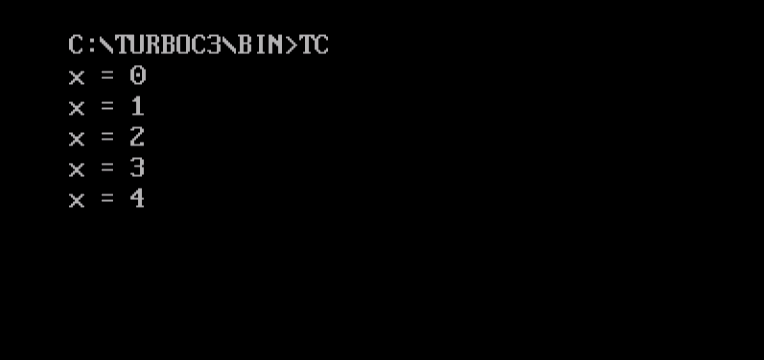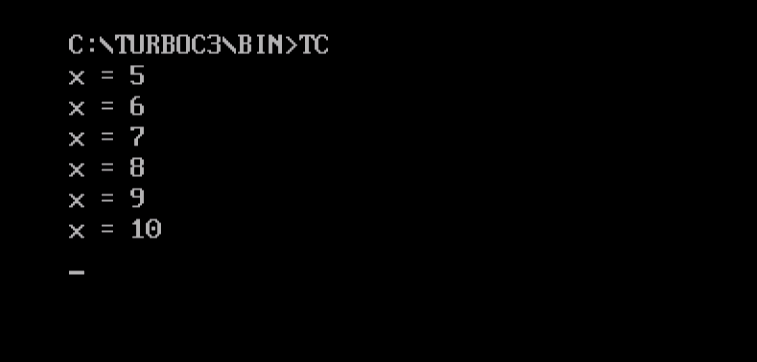# C++ Loops

## 30 Sep C++ Loops

C++ loops execute a block of code and this code executes while the condition is true. Here are the types of loops in C++,

Note: Learn how to run your first C++ program in Turbo C++. We will be running the following programs in the same way.

## C++ while loop

In while loop, the block of code executes only when the condition is true. It executes as long as the condition is true.

Here’s the syntax,

Here’s an example,

Here’s the output,## C++ dowhile loop

In do…while loop, the condition is checked at the bottom of the loop. Therefore, the loop executes at least once.

Here’s the syntax:

Here’s an example,

Here’s the output,## C++ for loop

It is used when you can set how many times a statement is to be executed.

Here’s the syntax,

Here’s an example,

Here you can see iterations from x = 5 to x = 10,

Here’s the output,[email protected]

We work to create free tutorials for all.